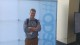# xgboost time series forecast in R

## 8 thoughts on “xgboost time series forecast in R”

•Nick says:

Looking into predicting stocks. I have tried out the prophet package for time series which is good. Had no luck with adding regressors. This example is definitely helpful. Do you have any other suggestions on predicting time series with regressors? I know you mentioned arima, but wondering if you had other suggestions.

•Joel says:

Why does the forecast period (blue) look like a copy of the last observed period (black)?

•Alice says:

Because the data was randomly generated. I changed the data to make example more meaningful.

•Abhinav says:

Does forecasting even if the data is less than 1 year into history?

•Alice says:

in principle yes, depends on data granularity and of course the more points the more sensible prediction may be expected.

•Panos says:

Is there an easy way to convert this solution to be working on weekly data (or even daily) ??
I have tried changing the frequency of the ts objects (created in the fitted and xgb_forecast variables) to a weekly frequency or even tried to convert the xts objects to a ts without considering a freq but had no luck…

•Alice says:

Yes! The key is to specify your artificial time related columns properly. If daily data – daily granularity, if weekly data – weekly granularity ect.

For some daily dataset:
extended_data_mod <- extended_data %>%
dplyr::mutate(.,
days = lubridate::day(Date),
months = lubridate::month(Date),
years = lubridate::year(Date))

Then for the fitted values and prediction you need to pass daily index:
fitted <- xgb_model %>%
stats::predict(x_train) %>%
stats::ts(start = c(lubridate::year(min(train\$Date)), lubridate::yday(min(train\$Date))),
end = c(lubridate::year(max(train\$Date)), lubridate::yday(max(train\$Date))),
frequency = 365)

xgb_forecast <- xgb_pred %>%
stats::ts(start = c(lubridate::year(min(pred\$Date)), lubridate::yday(min(pred\$Date))),
end = c(lubridate::year(max(pred\$Date)), lubridate::yday(max(pred\$Date))),
frequency = 365)

Similarly for any other data granularity.

•Vladimir says:

I have an error every time in this part:
xgb_model = train(
x = x_train,
y = y_train,
trControl = xgb_trcontrol,
tuneGrid = xgb_grid,
method = “xgbTree”,
error: Error in `[.xgb.DMatrix`(x, 0, , drop = FALSE) :